# Recursion

A method of defining functions studied in the theory of algorithms and other branches of mathematical logic. This method has been used for a long time in arithmetic to define sequences of numbers (progressions, Fibonacci numbers, etc.). Recursion plays an important role in computational mathematics (recursive methods). Finally, in set theory transfinite recursion is often used.

For a long time the term "recursion" was used by mathematicians without being accurately defined. Its approximate intuitive sense can be described in the following way: The value of a sought functionat an arbitrary point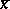(by point is understood a tuple of values of arguments) is determined, generally speaking, by way of the values of this same function at other pointsthat in a sense "precede". (The very word "recur" means "return" .) At certain "initial" points the values ofmust of course be defined directly. Sometimes recursion is used to define several functions simultaneously; then the above-mentioned definitions are taken with a corresponding modification. Examples of different kinds of recursion will be given below. The relation "x1 precedes x2" (where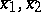belong to the domain of the sought function) in various types of recursion ( "recursive schemes" ) may have a different sense. It must, however, be "well-founded recursionwell-founded" (i.e. there should not be an infinite sequence of points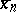,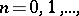such that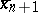precedes). Furthermore, it is implicitly understood that the relation is "sufficiently natural" (e.g. it is desirable that this relation be seen from the actual description of a recursive scheme and not from the process of its application). This last condition has a purely heuristic value (e.g. for determining any special, comparatively simple type of recursion). A more accurate definition of this condition is essentially inseparable from a more accurate description of the concept of recursion itself, and for this it is essential to establish which type of formal expressions can be acknowledged as recursive definitions.

Where it is a question of recursive descriptions of numerical functions (i.e. functions with natural arguments and natural values), it is usually implied that such descriptions define a way of computing the functions being determined. Here and in the sequel (except in the concluding remarks), the term "recursion" will be understood in precisely this sense. The simplest and most widely used recursive scheme is primitive recursion: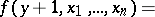where the functionsandare assumed to be known,is the function to be determined,is a variable according to which the recursion is conducted, andare parameters not participating in the recursion. The closest generalization of this scheme is the so-called course-of-value recursion, which includes those types of recursive definitions in which, as in primitive recursion, only one variable participates in the recursion. The corresponding relation of precedence coincides with the normal ordering of the natural numbers (sometimes, however, this term is used in an even wider sense). The most typical form of course-of-value recursion is as follows: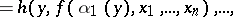where,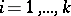. Mathematical logic often involves primitive recursive functions, i.e. functions that can be obtained after a finite number of steps using substitution and primitive recursion, starting from a specific fixed supply of basic functions (e.g.,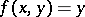, etc.). A sequence of functional equalities that describes such a structure is called a primitive recursive description of the corresponding function. These descriptions are syntactic objects (i.e. chains of symbols) possessing a definite effectively recognizable structure. Practically all numerical functions used in mathematics for some concrete purpose prove to be primitive recursive. This largely explains the interest there is in this class of functions.

More complex types of recursive definitions are obtained when the recursion occurs simultaneously over several variables. These definitions, as a rule, lead out of the class of primitive recursive functions, although the corresponding relation of precedence may be completely natural. For example, the values of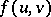may participate in the definition of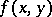, whereor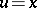,, as this occurs in the following scheme of double recursion: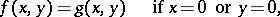This scheme has not yet been reduced to primitive recursion. On the other hand, a lot of more involved recursive definitions have been reduced to it (see ).

The partial types of recursion referred to have precise mathematical definitions, as opposed to the vague "near mathematical" ideas about "recursion in general" . The refinement of these ideas is naturally thought of as a search for a suitable algorithmic language (i.e. a formal language for describing computable procedures) that incorporates all the imaginable types of recursion without being too broad. One can rightly expect that the development of such a refinement may require further additional agreements, which bring something new to the intuitive understanding of recursion. In this connection it is not without interest to note that all the recursive schemes examined above are oriented towards the generation of total (everywhere defined) functions. Thus, if in a scheme of primitive recursion the functions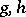are total, then so is. In general, recursive definitions that define partial functions intuitively look rather unnatural. However, such recursions are brought into the analysis for a definite reason, connected with the so-called diagonal method. Thus, let there be given an algorithmic languagein which only total functions are defined, and let the syntactic structure ofnot extend beyond the limits of an intuitively understood recursiveness. It is of course implied that expressions in the language(being descriptions of functions) are algorithmically recognizable and that there is a uniform method of computing functions that can be represented in, according to their descriptions. If expressions inare regarded as being simply chains of symbols, then each of these chains can be considered as a representation of a natural number in a suitable number system, the "code" of the given expression. Now, let the function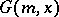be defined as follows: Ifis the code for describing (in) a certain one-place function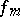, then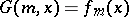. Otherwise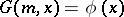, whereis some fixed function representable in. It is clear thatis computable (cf. Computable function) and total, as is the function. Butis not expressible in, since for anythe equality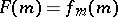is impossible. (This argument makes considerable use of the totality of.) The question arises: Is the given description ofrecursive? Ifis taken to be the language of primitive recursive descriptions, then the description turns out to be reducible to a double recursion. Generally, the situation is unclear because of the vagueness in intuitive ideas about recursion, so that a positive answer to this question appears to be an additional agreement. If it is accepted (as is implicitly done in contemporary logic), then the existence of a languagethat describes in detail all types of "total" recursion proves to be impossible.

Meanwhile, if partial functions can also be expressed in(and if their descriptions are acknowledged to be recursive), then diagonalization does not necessarily extend beyond, so that this language may be suitable for an adequate precization of recursiveness. It is true that this involves a certain re-thinking of the very concept of recursion, and the contemporary strict definition of this concept is not fully in line with previously held intuitive ideas. In the new refined sense, recursion is a specific syntactic operation (with a fixed interpretation) used to construct expressions in various algorithmic languages. Ifis one of these languages, it is natural to assume that there are other syntactic operations in it on which the concrete type of recursive descriptions independs. Generally speaking, expressions indo not necessarily describe only numerical functions. Several of them may define functional operators and other objects. This is necessary, in particular, for defining recursion. Recursive descriptions inare systems of functional equations of the type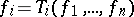,, where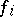are the functions being defined and the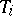are expressions indefining a specific operator in a collection. But all expressible operators inmust be effective (sinceis an algorithmic language) and hence monotone (i.e. they preserve the relation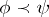, which means thatsupplements the definition of). Because of this, every system of the given type has a minimal (in the sense of the relation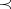) solution and, according to the definition, is a recursive description of the functions that make up this solution. Starting from the given description, the sought minimal solution can be obtained by means of the following process. For, let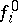be a nowhere-defined function and let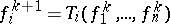be such that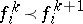. It is easy to verify that the sought functionsare obtained by "combination" of the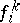. Methods for a simultaneous computation ofare defined in the same way. This process defines a more or less natural relation of precedence on arguments, which also justifies (to a satisfactory extent) the use of the term "recursion" in this connection.

In order to include the aforementioned recursive schemes in this definition, it is essential that the languagebe not too poor. Thus, already in the case of primitive recursion, substitutions and a "piecewise" definition have been required (i.e. a definition of the function by several equalities). Furthermore, these two syntactic operations, in combination with the recursion just defined, are already sufficient to obtain all computable functions (starting from the basic ones, cf. Computable function). With appropriate assumptions about, one can confidently claim that the given definition of recursion includes all intuitively conceivable recursive descriptions. At the same time, the general definition given has the characteristic properties of an informally understood recursiveness that are acknowledged to be fundamental in modern mathematics.

The fruitfulness of the given definition of recursion consists not only in its significance in the theory of algorithms, but also in that it permits one to look from an "algorithmic" (in the generalized sense) point of view at several structures of abstract mathematics that have a definite similarity with "numerical" recursions (transfinite recursion, inductive definition, recursive hierarchies, etc.).

How to Cite This Entry:
Recursion. N.V. Belyakin (originator), Encyclopedia of Mathematics. URL: http://encyclopediaofmath.org/index.php?title=Recursion&oldid=11609
This text originally appeared in Encyclopedia of Mathematics - ISBN 1402006098